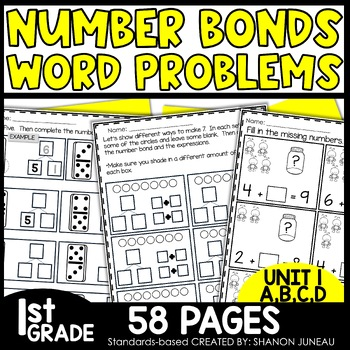1st, Homeschool
Subjects
Standards
Resource Type
Formats Included
• PDF
•Activity
Pages
57 pages
Easel Activity Included
This resource includes a ready-to-use interactive activity students can complete on any device. Easel by TpT is free to use! Learn more.
##### Also included in
1. This amazing year-long bundle has everything you need to make sure your students are grasping the 1st grade concepts they need to be mathematicians! These NO PREP worksheets can also be used with EASEL digital and are the perfect addition to your first grade curriculum. They cover all first grade
\$50.00
\$67.98
Save \$17.98
2. These print and go math worksheets are perfect for math centers, independent practice, sub tubs, and assessments. This Math Bundle covers:missing addends simple word problems using pictures number bonds using 10, 9, 8, 7 as the whole, counting on, and finding the sumcommutative property of addition
\$12.20
\$15.25
Save \$3.05

### Description

️These Missing Addends 1st Grade Math Worksheets are a great addition to your first grade print and go resources. Students will apply skills to become fluent with missing addends, word problems using pictures, number bonds, and counting on. These worksheets are great for introducing a concept, review, assessments, homework, or center practice.

BUNDLED FOR CONVENIENCE

These worksheets cover:

• simple word problems using pictures
• number bonds using 10, 9, 8, 7 as the whole
• counting on
• finding the sum

OTHER RESOURCES: If you are looking for other resources to help with unit 1, here are my money saving bundles:

Main Standards Covered:

• 1.OA.A.1 Use addition and subtraction within 20 to solve word problems involving situations of adding to, taking from, putting together, taking apart, and comparing, with unknowns in all positions, e.g., by using objects, drawings, and equations with a symbol for the unknown number to represent the problem.
• 1.OA.C.5 Relate counting to addition and subtraction (e.g., by counting on 2 to add 2).
• 1.OA.C.6 Add and subtract within 20, demonstrating fluency for addition and subtraction within 10. Use strategies such as counting on; making ten (e.g., 8 + 6 = 8 + 2 + 4 = 10 + 4 = 14); decomposing a number leading to a ten (e.g., 13 - 4 = 13 - 3 - 1 = 10 - 1 = 9); using the relationship between addition and subtraction (e.g., knowing that 8 + 4 = 12, one knows 12 - 8 = 4); and creating equivalent but easier or known sums (e.g., adding 6 + 7 by creating the known equivalent 6 + 6 + 1 = 12 + 1 = 13).
• 1.OA.D.8 Determine the unknown whole number in an addition or subtraction equation relating three whole numbers. For example, determine the unknown number that makes the equation true in each of the equations 8 + ? = 11, 5 = _ - 3, 6 + 6 = _.

WHO LIKES A DEAL?

• Follow me and be notified when new products are uploaded. New products are always 50% off for the first 24 hours they are posted (except for resources that are under \$1.50). It pays to follow me!

QUESTIONS OR CONCERNS

I sure appreciate you!

Shanon Juneau ❤️

We Are Better Together

Total Pages
57 pages
N/A
Teaching Duration
N/A
Report this Resource to TpT
Reported resources will be reviewed by our team. Report this resource to let us know if this resource violates TpT’s content guidelines.

### Standards

to see state-specific standards (only available in the US).
Use addition and subtraction within 20 to solve word problems involving situations of adding to, taking from, putting together, taking apart, and comparing, with unknowns in all positions, e.g., by using objects, drawings, and equations with a symbol for the unknown number to represent the problem.
Relate counting to addition and subtraction (e.g., by counting on 2 to add 2).
Add and subtract within 20, demonstrating fluency for addition and subtraction within 10. Use strategies such as counting on; making ten (e.g., 8 + 6 = 8 + 2 + 4 = 10 + 4 = 14); decomposing a number leading to a ten (e.g., 13 - 4 = 13 - 3 - 1 = 10 - 1 = 9); using the relationship between addition and subtraction (e.g., knowing that 8 + 4 = 12, one knows 12 - 8 = 4); and creating equivalent but easier or known sums (e.g., adding 6 + 7 by creating the known equivalent 6 + 6 + 1 = 12 + 1 = 13).
Determine the unknown whole number in an addition or subtraction equation relating three whole numbers. For example, determine the unknown number that makes the equation true in each of the equations 8 + ? = 11, 5 = â¯ - 3, 6 + 6 = â¯.